# DC Field I(V) Curve

This routine aggregates the I(V) curves from individual modules, and then sums them in parallel and in series to yield an I(V) curve profile that is representative of the entire DC Field. Figure 37 shows a general schematic of such parallel-series connection.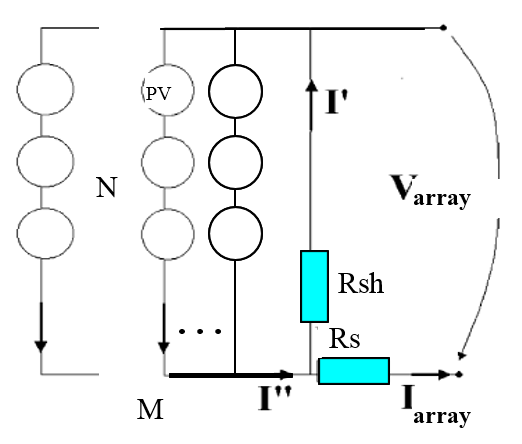### Figure 37. Array Comprised of N Series x M Parallel PV Modules

Currents add for parallel-connected modules and voltages add in series-connected modules.

## Inputs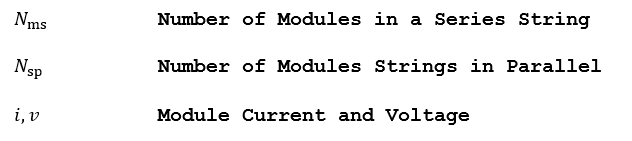## Outputs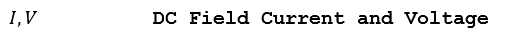## Algorithm

1.) For combination of identical modules in series and parallel, the points on the I(V) curves or current and voltages can be scaled as follows:

a.) Compute the aggregate I(V) curve for a series string comprised of Nms identical modules. The voltages can be multiplied by the number of modules in the series string; the current remains unchanged: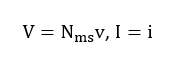b.) Compute the I(V) curve for a parallel connection of Nsp identical series strings in parallel modules. The currents can be multiplied by the number of strings in parallel; the voltage remains unchanged: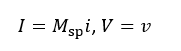2.) For heterogeneous arrays comprised of modules that do not exhibit the same I(V) curve (i.e. a cool shaded module connected to a hot unshaded module), the individual I(V) curves must be added:

a.) Parallel connection: Combine heterogeneous modules or strings connected in parallel by interpolating the I(V) array at common voltage points so that the sampled currents are aligned; then sum the individual currents.

b.) Series connection: Combine heterogeneous modules or strings connected in series by interpolating the I(V) array at common current points so that the sampled voltages are aligned; then sum the individual voltages.